# Obtuse Angle - Definition with Examples

The Complete K-5 Math Learning Program Built for Your Child

• 30 Million Kids

Loved by kids and parent worldwide

• 50,000 Schools

Trusted by teachers across schools

• Comprehensive Curriculum

Aligned to Common Core

What is obtuse Angle?

An obtuse angle is a type of angle that is always larger than 90° but less than 180°. In other words, it lies between 90° and 180°.

In the given figure, ∠XYZ shows an obtuse angle.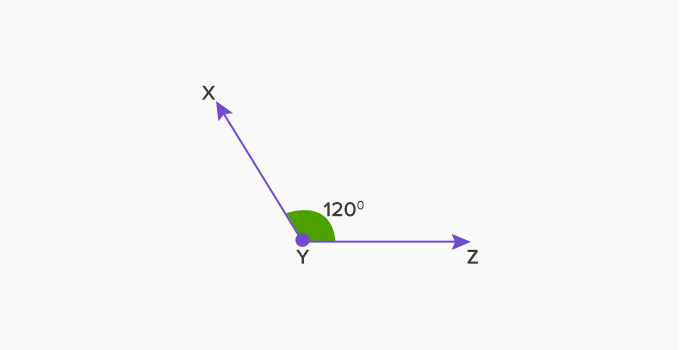It is an obtuse angle as it measures more than 90 degrees as shown: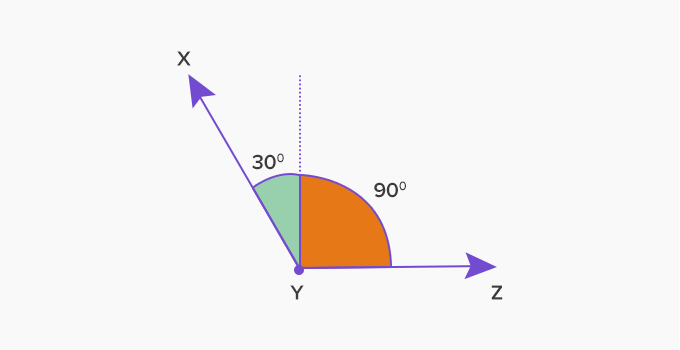Some more examples of obtuse angle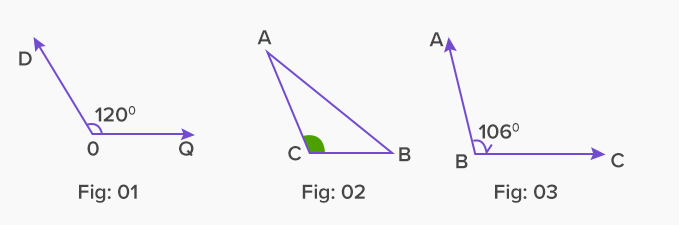Here, in Fig: 01, ∠DOQ forms an obtuse angle. And, in Fig: 02 and Fig: 03 ∠ACB  and ∠ABC form obtuse angles respectively.

Obtuse angle in polygons: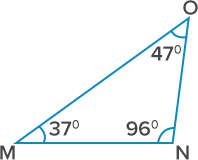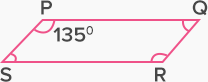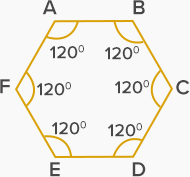In triangle MNO, ∠N is an obtuse angle. In parallelogram PQRS, ∠P is an obtuse angle. In this hexagon, all angles are obtuse.

In the given polygon ABCDEF, can you tell which angles are obtuse and which are not? What do you think about ∠x ?Here, ∠x  is an obtuse angle with a measure of 168°

Real life examples of obtuse angles: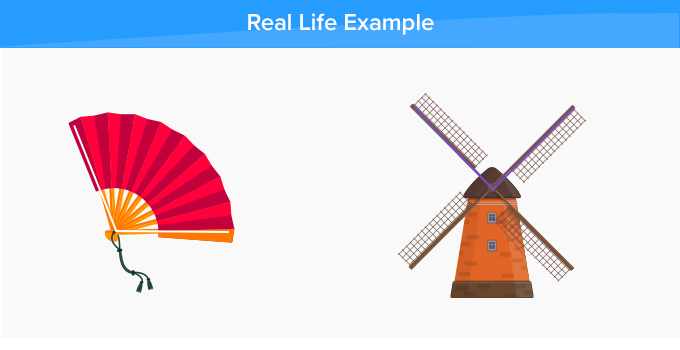It is important to have a clear idea about the angle formation to understand the obtuse angle.

Drawing an obtuse angle

We know that when two rays meet at a point, they form an angle. The two rays are called the arms of the angle, and the point is called the vertex.

Let us learn the steps to draw an obtuse angle using a protractor: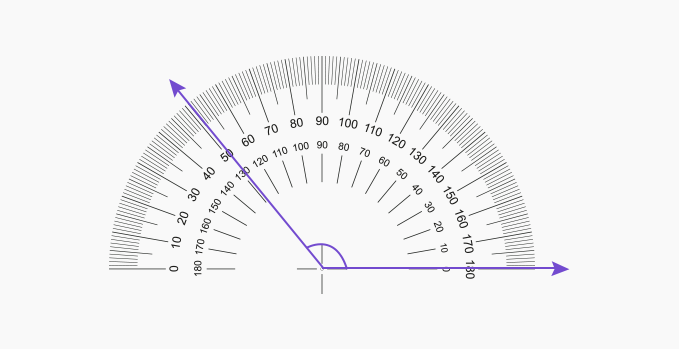Won Numerous Awards & Honors